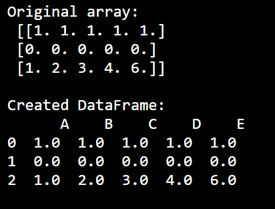# How to turn a 3d numpy array into a pandas dataframe of numpy 1d arrays?

Given a 3d NumPy array, we have to turn it into a pandas dataframe of NumPy 1d arrays.
Submitted by Pranit Sharma, on December 31, 2022

NumPy is an abbreviated form of Numerical Python. It is used for different types of scientific operations in python. Numpy is a vast library in python which is used for almost every kind of scientific or mathematical operation. It is itself an array which is a collection of various methods and functions for processing the arrays.

## Problem statement

We need to create a pandas dataframe off of it which would have as many rows as the array's 1st dimension's size and the number of columns would be the size of the 2nd dimension. The values of the dataframe should be the actual vectors (numpy arrays) with the size of the third dimension.

## Turning a 3d numpy array into a pandas dataframe of numpy 1d arrays

For this purpose, we will use the pandas.DataFrame.from_records() method inside which we will pass the array.

Let us understand with the help of an example,

## Python program to turn a 3d numpy array into a pandas dataframe of numpy 1d arrays

```# Import numpy
import numpy as np

# Import pandas
import pandas as pd

# Creating an array
arr = np.array([
[ 1.,  1.,  1.,  1.,  1.],
[ 0.,  0.,  0.,  0.,  0.],
[ 1.,  2.,  3.,  4.,  6.]
])

# Display original array
print("Original array:\n",arr,"\n")

# Creating a dataframe with array
df = pd.DataFrame(arr, columns=['A', 'B', 'C', 'D', 'E'])

# Display dataframe
print("Created DataFrame:\n",df,"\n")
```

## Output

The output of the above program is: